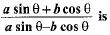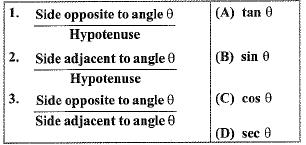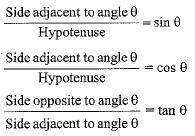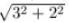Courses

# MCQ : Introduction To Trigonometry - 1

## 10 Questions MCQ Test Mathematics (Maths) Class 10 | MCQ : Introduction To Trigonometry - 1

Description
This mock test of MCQ : Introduction To Trigonometry - 1 for Class 10 helps you for every Class 10 entrance exam. This contains 10 Multiple Choice Questions for Class 10 MCQ : Introduction To Trigonometry - 1 (mcq) to study with solutions a complete question bank. The solved questions answers in this MCQ : Introduction To Trigonometry - 1 quiz give you a good mix of easy questions and tough questions. Class 10 students definitely take this MCQ : Introduction To Trigonometry - 1 exercise for a better result in the exam. You can find other MCQ : Introduction To Trigonometry - 1 extra questions, long questions & short questions for Class 10 on EduRev as well by searching above.
QUESTION: 1

### The value of (sin 30° + cos 30°) - (sin 60° + cos 60°) is

Solution:

sin 30° = 1/2,
cos 30°=√3/2,
sin 60°=√3/2,
cos 60°=1/2,
By putting the value of sin 30°, cos 30°, sin 60° and cos 60° in equation

We get=
(sin30°+cos30°)-(sin60°+cos60°)=(1/2+√3/2)-(√3/2+1/2)
=0

QUESTION: 2

### Ratios of sides of a right triangle with respect to its acute angles are known as

Solution:

The ratios of sides of a right-angled triangle with respect to any of its acute angles are known as the trigonometric ratios of that particular angle.

QUESTION: 3

### The value of (sin 45° + cos 45°) is

Solution:

Since, sin 45°= 1/√2 and cos 45°= 1/√2
Therefore, sin 45° + cos 45° = 1/√2 + 1/√2 = 2/√2 = √2

QUESTION: 4

If tan θ = a/b then the value ofSolution:

Let,angle= θ
(asinθ + bcosθ)/(asinθ - bcosθ)
Dividing both numerator and denominator from cosθ
We get,
atanθ +b/atanθ - b
= ( a.a/b + b) /(a.a/b - b) =(a²/b +b)/(a²/b - b)
=(a² + b²/a²- b²)

QUESTION: 5

If 6cotθ + 2cosecθ = cotθ + 5cosecθ, then cosθ is

Solution:

6cot+2cosec=cot+5cosec
6cot-cot=5cosec-2cosec
5cot=3cosec
5cos/sin=3/sin
cos=3/5

QUESTION: 6

Match the Columns:Solution:QUESTION: 7

9 sec2 A - 9tan2 A is equal to

Solution:

9 sec2 A - 9 tan2 A
= 9( sec2 A - tan2 A)
= 9 × 1
= 9

QUESTION: 8

The value of sin2 30° - cos2 30° is

Solution:

Solution :- sin^2 30° − cos^2 30°

= (1/2)2 −((3)1/2/2)2

= 1/4 - 3/4

= -1/2

QUESTION: 9

If tan A = 3/2, then the value of cos A is

Solution:

Tanθ = Perpendicular / Base
We are given that TanA = 3/2
On comparing
Perpendicular = 3
Base = 2
To fing hypotenuse
Hypotenuse2 = Perpendicular2 + Base2
Hypotenuse2 = 32 + 22
Hypotenuse =Hypotenuse = 3.6

Cosθ = Base / Hypotenuse
CosA = 2 / 3.6
Hence the value of Cos A is 2/3.6=2/√13

QUESTION: 10

If 3 cot θ = 2, then the value of tan θ

Solution:

3 cot θ = 2 ⇒ cot θ = 2/3 ⇒ tan θ = 3/2